# NCERT Solutions class-11 Maths Exercise 6.2## myCBSEguide App

CBSE, NCERT, JEE Main, NEET-UG, NDA, Exam Papers, Question Bank, NCERT Solutions, Exemplars, Revision Notes, Free Videos, MCQ Tests & more.

Exercise 6.2

Solve the following inequalities graphically in two dimensional planes:

1.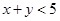Ans. Given:Table of values satisfying the equation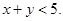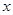1 2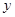4 3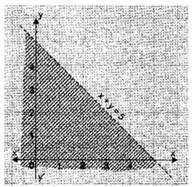Putting (0, 0) in the given in equation,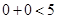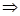0 < 5

which is true.

Therefore, Half plane oftowards origin.

2.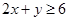Ans. Given:Table of values satisfying the equation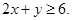1 24 2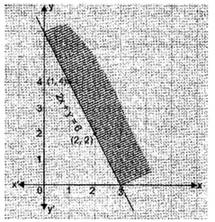Putting (0, 0) in the given in equation,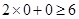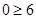which is false.

Therefore, Half plane ofaway from the origin.

3.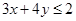Ans. Given:Table of values satisfying the equation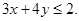0 43 0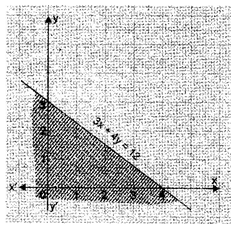Putting (0, 0) in the given in equation,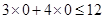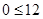which is true.

Therefore, Half plane oftowards the origin.

4.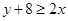Ans. Given:Table of values satisfying the equation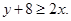5 62 4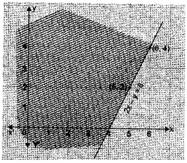Putting (0, 0) in the given in equation,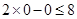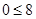which is true.

Therefore, Half plane oftowards the origin.

5.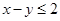Ans. Given:Table of values satisfying the equation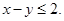2 31 2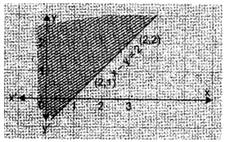Putting (0, 0) in the given in equation,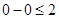which is true.

Therefore, Half plane oftowards the origin.

6.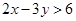Ans. Given:Table of values satisfying the equation6 92 4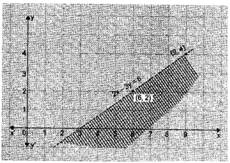Putting (0, 0) in the given in equation,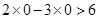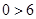which is false.

Therefore, Half plane ofaway from the origin.

7.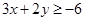Ans. Given:Table of values satisfying the equation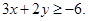2 00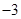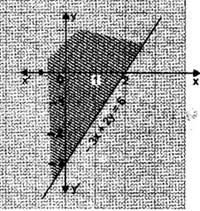Putting (0, 0) in the given in equation,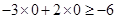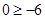which is true.

Therefore, Half plane oftowards the origin.

8.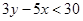Ans. Given:Table of values satisfying the equation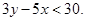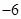00 10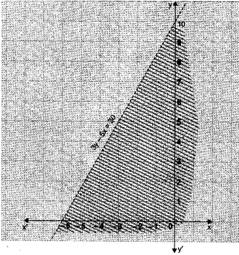Putting (0, 0) in the given in equation,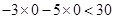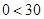which is true.

Therefore, Half plane oftowards the origin.

9.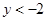Ans. Given:Table of values satisfying the equation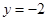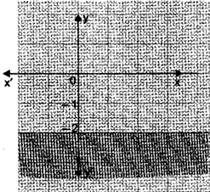Putting (0, 0) in the given in equation,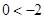which is false.

Therefore, Half plane ofaway from the origin.

10.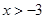Ans. Given: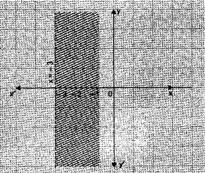Putting (0, 0) in the given in equation,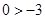which is true, therefore, Half plane oftowards the origin.### 4 thoughts on “NCERT Solutions class-11 Maths Exercise 6.2”

1. Nice
Solution

2. Very nice solution